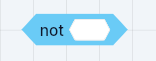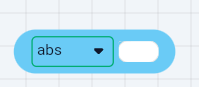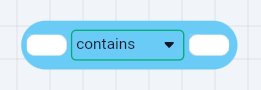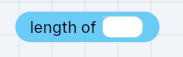# Operator Block

Tink Logic provides blocks for Comparison, Logical, and Mathematical operations. Operations on values become easier with Operator blocks. Here is a list of Operator blocks.

• `Comparison operator` compares two values that are equal or not equal. If values are not equal, it checks if one value is greater than or less than the other value. This is a Boolean block that results in a true or false value.• `Mathematical operator` performs mathematical operations like addition, subtraction, multiplication, and division on two values.• Invert block inverts a value. If the value is true, it becomes false, and vice versa.• `Logical operator` performs AND/OR operation on two values. It can also generate a random number between two values.• `Trigonometric operator` carries out trigonometric functions like calculating cos, tan, square root, absolute value, and modulus of a number. It also does the rounding of a number.• `Text operator` operates on Text values. It checks if a text value contains another text value. It also joins two text values. E.g. A Join B produces AB. It gets a Text character at a given position in a longer text value• `Length operator` returns the number of characters in a String or number of items in a List.• `JSON operator` performs GET / SET operations on a JSON string. In the GET operation, this block accepts a JSONPointer string and allows you to access the data that matches. In the SET operation, it accepts a JSONPointer string and a value. It will replace the data matching the pointer or a create new one if it doesn't exist. It does not modify the original object. But it returns the modified copy.